# Basic Concepts In Geometry Class 9th Mathematics Part Ii MHB Solution

###### Practice Set 1.1
Question 1.

Find the distances with the help of the number line given below.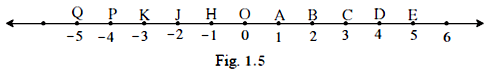i. d(B, E)

ii. d(j, A)

iii. d(P, C)

iv. d(J, H)

v. d(K, O)

vi. d(O, E)

vii. d(Q, B)

To find the distance between two points, consider their co-ordinates and subtract smaller co-ordinate from the larger.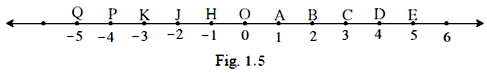(i) Now, the co-ordinates of B and E are 2 and 5 respectively. We know that 5 > 2.

So, d (B, E) = 5 – 2 = 3

⇒ d (B, E) = 3

(ii) The co-ordinates of J and A are -2 and 1 respectively. We know that 1 > -2.

So, d (J, A) = 1 – (-2) = 1 + 2 = 3

⇒ d (J, A) = 3

(iii) The co-ordinates of P and C are -4 and 3 respectively. We know that 3 > -4.

So, d (P, C) = 3 – (-4) = 3 + 4 = 7

⇒ d (P, C) = 7

(iv) The co-ordinates of J and H are -2 and -1 respectively. We know that -1 > -2.

So, d (J, H) = (-1) – (-2) = -1 + 2 = 1

⇒ d (J, H) = 1

(v) The co-ordinates of K and O are -3 and 0 respectively. We know that 0 > -3.

So, d (K, O) = 0 – (-3) = 0 + 3 = 3

⇒ d (K, O) = 3

(vi) The co-ordinates of O and E are 0 and 5 respectively. We know that 5 > 0.

So, d (O, E) = 5 – 0 = 5

⇒ d (O, E) = 5

(vii) The co-ordinates of P and J are -4 and -2 respectively. We know that -2 > -4.

So, d (P, J) = (-2) – (-4) = -2 + 4 = 2

⇒ d (P, J) = 2

(viii) The co-ordinates of Q and B are -5 and 2 respectively. We know that 2 > -5.

So, d (Q, B) = 2 – (-5) = 2 + 5 = 7

⇒ d (Q, B) = 7

Question 2.

If the co-ordinate of A is x and that of B is y, find d(A, B).

i. x = 1, y = 7

ii. x = 6, y = -2

iii. x = -3, y = 7

iv. x = -4, y = -5

v. x = -3, y = -6

vi. x = 4, y = -8

To find the distance between two points, consider their co-ordinates and subtract smaller co-ordinate from the larger.

(i) The co-ordinates of A and B are 1 and 7 respectively. We know that 7 > 1.

So, d (A, B) = 7 – 1 = 6

⇒ d (A, B) = 6

(ii) The co-ordinates of A and B are 6 and -2 respectively. We know that 6 > -2.

So, d (A, B) = 6 – (-2) = 6 + 2 = 8

⇒ d (A, B) = 8

(iii) The co-ordinates of A and B are -3 and 7 respectively. We know that 7 > -3.

So, d (A, B) = 7 – (-3) = 7 + 3 = 10

⇒ d (A, B) = 10

(iv) The co-ordinates of A and B are -4 and -5 respectively. We know that -4 > -5.

So, d (A, B) = (-4) – (-5) = -4 + 5 = 1

⇒ d (A, B) = 1

(v) The co-ordinates of A and B are -3 and -6 respectively. We know that -3 > -6.

So, d (A, B) = (-3) – (-6) = -3 + 6 = 3

⇒ d (A, B) = 3

(vi) The co-ordinates of A and B are 4 and -8 respectively. We know that 4 > -8.

So, d (A, B) = 4 – (-8) = 4 + 8 = 12

⇒ d (A, B) = 12

Question 3.

From the information given below, find which of the point is between the other two. If the points are not collinear, state so.

i. d (P, R) = 7, d (P, Q) = 10, d (Q, R) = 3

ii. d (R, S) = 8, d (S, T) = 6, d (R, T) = 4

iii. d (A, B) = 16, d (C, A) = 9, d (B, C) = 7

iv. d (L, M) = 11, d (M, N) = 12, d (N, L) = 8

v. d (X, Y) = 15, d (Y, Z) = 7, d (X, Z) = 8

vi. d (D, E) = 5, d (E, F) = 8, d (D, F) = 6

For any three-distinct collinear points P, Q and R, the point Q is said to be in between P and R if

d (P, Q) + d (Q, R) = d (P, R).

(i) Given: d (P, R) = 7, d (P, Q) = 10, d (Q, R) = 3

Now, consider d (P, Q) = 10 ……….(I)

And d (P, R) + d (Q, R) = 7 + 3 = 10 ……….(II)

∴ Using (I) and (II), we have

d (P, R) + d (Q, R) = d (P, Q)

⇒ R lies between P and Q.

(ii) Given: d (R, S) = 8, d (S, T) = 6, d (R, T) = 4

Here, d (R, S) + d (S, T) = 8 + 6 = 14 ≠ 4 = d (R, T)

Also, d (R, S) + d (R, T) = 8 + 4 = 12 ≠ 6 = d (S, T)

And d (S, T) + d (R, T) = 6 + 4 = 10 ≠ 8 = d (R, S)

Hence, the points R, S and T are non-collinear.

(iii) Given: d (A, B) = 16, d (C, A) = 9, d (B, C) = 7

Now, consider d (A, B) = 16 ……….(I)

And d (C, A) + d (B, C) = 9 + 7 = 16 ……….(II)

∴ Using (I) and (II), we have

d (C, A) + d (B, C) = d (A, B)

⇒ C lies between A and B.

(iv) Given: d (L, M) = 11, d (M, N) = 12, d (N, L) = 8

Here, d (L, M) + d (M, N) = 11 + 12 = 23 ≠ 8 = d (N, L)

Also, d (L, M) + d (N, L) = 11 + 8 = 19 ≠ 12 = d (M, N)

And d (M, N) + d (N, L) = 12 + 8 = 20 ≠ 11= d (L, M)

Hence, the points L, M and N are non-collinear.

(v) Given: d (X, Y) = 15, d (Y, Z) = 7, d (X, Z) = 8

Now, consider d (X, Y) = 15 ……….(I)

And d (Y, Z) + d (X, Z) = 7 + 8 = 15 ……….(II)

∴ Using (I) and (II), we have

d (Y, Z) + d (X, Z) = d (X, Y)

⇒ Z lies between X and Y.

(vi) Given: d (D, E) = 5, d (E, F) = 8, d (D, F) = 6

Here, d (D, E) + d (E, F) = 5 + 8 = 13 ≠ 6 = d (D, F)

Also, d (D, E) + d (D, F) = 5 + 6 = 11 ≠ 8 = d (E, F)

And d (E, F) + d (D, F) = 8 + 6 = 14 ≠ 5 = d (D, E)

Hence, the points D, E and F are non-collinear.

Question 4.

On a number line, points A, B and C are such that d (A, C) = 10, d (C, B) = 8 Find d(A, B) considering all possibilities.

We know that the distance between two points is obtained by subtracting the smaller co-ordinate from the larger co-ordinate.

Given: d (A, C) = 10 and d (C, B) = 8

Now, we know that

If A, B and C are three distinct collinear points, then there are three possibilities:

(i) Point B is between A and C

(ii) Point C is between A and B

(iii) Point A is between B and C

Now, let (i) holds true, i.e. Point B is between A and C, then

d (A, B) + d (B, C) = d (A, C)

⇒ d (A, B) = d (A, C) – d (B, C) = 10 – 8 = 2

⇒ d (A, B) = 2

Next, let (ii) holds true, i.e. Point C is between A and B, then

d (A, C) + d (B, C) = d (A, B)

⇒ d (A, B) = 10 + 8 = 18

⇒ d (A, B) = 18

Lastly, let (iii) holds true, i.e. Point A is between B and C, then

D (A, B) + d (A, C) = d (B, C)

⇒ d (A, B) = d (B, C) – d (A, C) = 8 – 10 = -2

⇒ d (A, B) = -2, which is not possible as distance between any two points is a non-negative real number.

Hence, the value of d (A, B) is either 2 or 18.

Question 5.

Points X, Y, Z are collinear such that d (X, Y) = 17, d (Y, Z) = 8 find d(X, Z).

Given: X, Y and Z are collinear and

d (X, Y) = 17, d (Y, Z) = 8

We know that

If X, Y and Z are three distinct collinear points, then there are three possibilities:

(i) Point Y is between X and Z

(ii) Point Z is between X and Y

(iii) Point X is between Y and Z

Now, let (i) holds true, i.e. Point Y is between X and Z, then

d (X, Y) + d (Y, Z) = d (X, Z)

⇒ d (X, Z) = 17 + 8 = 25

⇒ d (X, Z) = 25

Next, let (ii) holds true, i.e. Point Z is between X and Y, then

d (X, Z) + d (Y, Z) = d (X, Y)

⇒ d (X, Z) = d (X, Y) - d (Y, Z) = 17 – 8 = 9

⇒ d (X, Z) = 9

Lastly, let (iii) holds true, i.e. Point X is between Y and Z, then

d (X, Y) + d (X, Z) = d (Y, Z)

⇒ d (X, Z) = d (Y, Z) – d (X, Y) = 8 – 17 = -9

⇒ d (X, Z) = -9, which is not possible as distance between any two points is a non-negative real number.

Hence, the value of d (X, Z) is either 25 or 9.

Question 6.

Sketch proper figure and write the answers of the following questions.

i. If A - B - C and /(AC) = 11, /(BC) = 6.5, then /(AB) =?

ii. If R - S - T and /(ST) = 3.7, /(RS) = 2.5, then /(RT) =?

iii. If X - Y - Z and /(XZ) = 3√7, /(XY) =√7, then /(YZ) =?

i. l (AC) = 11, l (BC) = 6.5We know that

For any three-distinct collinear points P, Q and R, the point Q is said to be in between P and R if

d (P, Q) + d (Q, R) = d (P, R), or

l (PQ) + l (QR) = l (PR)

So, we have l (AB) = l (AC) – l (BC) = 11 – 6.5 = 4.5

l (AB) = 4.5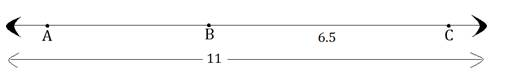ii. l (ST) = 3.7, l (RS) = 2.5

We know that

For any three-distinct collinear points P, Q and R, the point Q is said to be in between P and R if

d (P, Q) + d (Q, R) = d (P, R), or

l (PQ) + l (QR) = l (PR)

So, l (RT) = l (ST) + l (RS) = 3.7 + 2.5 = 6.2

l (RT) = 6.2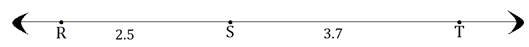iii.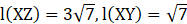We know that

For any three-distinct collinear points P, Q and R, the point Q is said to be in between P and R if

d (P, Q) + d (Q, R) = d (P, R), or

l (PQ) + l (QR) = l (PR)

So,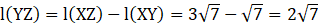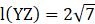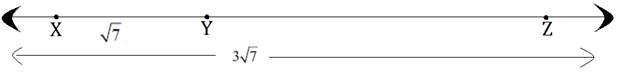Question 7.

Which figure is formed by three non-collinear points?

Three non- collinear points always form a Triangle.

###### Practice Set 1.2
Question 1.

The following table shows points on a number line and their co-ordinates. Decide whether the pair of segments given below the table are congruent or not.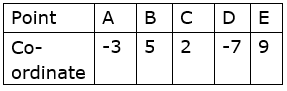i. seg DE and seg AB

ii. seg BC and seg AD

iii. seg BE and seg AD

Now, we know that

The distance between the end points of a segment is called the length of the segment and is denoted by l (AB) = seg AB = AB.

i. seg DE = l (DE) = 9 – (-7) = 9 + 7 = 16

and seg AB = l (AB) = 5 – (-3) = 5 + 3 = 8

Now, we know that

Two segments are congruent if the length of two segments is equal.

But, here, we have seg DE = 16 ≠ 8 = seg AB

So, seg DE and seg AB are not congruent.

ii. seg BC = l (BC) = 5 – 2 = 3

and seg AD = l (AD) = -3 – (-7) = -3 + 7 = 4

We know that

Two segments are congruent if the length of two segments is equal.

But, here, we have seg BC = 3 ≠ 4 = seg AD

So, seg BC and seg AD are not congruent.

iii. seg BE = l (BE) = 9 – 5 = 4

and seg AD = l (AD) = -3 – (-7) = -3 + 7 = 4

We know that

Two segments are congruent if the length of two segments is equal.

And, here, we have seg BE = 4 = seg AD

So, seg BE and seg AD are congruent.

Question 2.

Point M is the midpoint of seg AB. If AB = 8 then find the length of AM.

Since M is the midpoint of seg AB.

Therefore seg AM ≅ seg MB, i.e. seg AM = seg MB ……….(i)

Also, we know that

For any three distinct collinear points P, Q and R, the point Q is said to be in between P and R if

d (P, Q) + d (Q, R) = d (P, R), or

l (PQ) + l (QR) = l (PR)

So, seg AB = seg AM + seg MB

⇒ seg AB = 2 seg AM [Using (i)]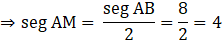So, seg AM = 4

Question 3.

Point P is the midpoint of seg CD. If CP = 2.5, find l(CD).

Since P is the midpoint of seg CD.

Therefore seg CP ≅ seg PD, i.e. seg CP = seg PD ……….(i)

Also, we know that

For any three-distinct collinear points P, Q and R, the point Q is said to be in between P and R if

d (P, Q) + d (Q, R) = d (P, R), or

l (PQ) + l (QR) = l (PR)

So, seg CD = seg CP + seg PD

⇒ seg CD = 2 seg CP [Using (i)]

⇒ seg CD = 2 × 2.5 = 5

So, seg CD = 5

Question 4.

If AB = 5 cm, BP = 2 cm and AP = 3.4 cm, compare the segments.

AB = 5 cm, BP = 2 cm and AP = 3.4 cm

Clearly, 2 < 3.4 < 5

So, BP < AP < AB.

Question 5.

Write the answers to the following questions with reference to figure 1.13.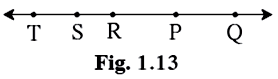i. Write the name of the opposite ray of ray RP.

ii. Write the intersection set of ray PQ and ray RP.

iii. Write the union set of ray PQ and ray QR.

iv. State the rays of which seg QR is a subset.

v. Write the pair of opposite rays with common end point R.

vi. Write any two rays with common end point S.

vii. Write the intersection set of ray SP and ray ST.

i. Ray RS or Ray RT

ii. Ray PQ

iii. Ray QR

iv. Ray RQ or Ray SQ or Ray TQ

v. Ray RT and Ray RQ

vi. Ray SQ, Ray SR or Ray SP

Vii. Point S

Question 6.

Answer the questions with the help of figure 1.14.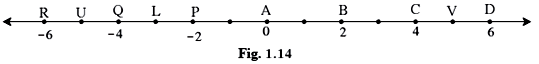i. State the points which are equidistant from point B.

ii. Write a pair of points equidistant from point Q.

iii. Find d(U, V), d(P, C), d(V, B), d(U, L).

i. Points A and C or Points P and D

ii. Points U and L or Points R and P

iii. d (U, V) = 5 – (-5) = 5 + 5 = 10

d (U, V) = 10

d (P, C) = 4 – (-2) = 4 + 2 = 6

d (P, C) = 6

d (V, B) = 5 – 2 = 3

d (V, B) = 3

d (U, L) = -3 – (-5) = -3 + 5 = 2

d (U, L) = 2

###### Practice Set 1.3
Question 1.

Write the following statements in ‘if-then’ form.

i. The opposite angles of a parallelogram are congruent.

ii. The diagonals of a rectangle are congruent.

iii. In an isosceles triangle, the segment joining the vertex and the mid-point of the base is perpendicular to the base.

The if-then forms for the above statements are as follows:

i. If a quadrilateral is a parallelogram then its opposite angles are congruent.

ii. If a quadrilateral is a rectangle then its diagonals are congruent.

iii. If a triangle is isosceles then the segment joining the vertex of the triangle and the midpoint of the base is perpendicular to the base.

Question 2.

Write converses of the following statements.

i. The alternate angles formed by two parallel lines and their transversal are congruent.

ii. If a pair of the interior angles made by a transversal of two lines are supplementary then the lines are parallel.

iii. The diagonals of a rectangle are congruent.

We know that if the antecedent (if part) and consequent (then part) in a given conditional statement are interchanged, then the resulting statement is called the Converse.

So, the converses for the above statements are as follows:

i. If the alternate angles made by two lines and its transversal are congruent then the lines are parallel.

ii. If two parallel lines are intersected by a transversal then the interior angles so formed are supplementary.

iii. If the diagonals of a quadrilateral are congruent then the quadrilateral is a rectangle.

###### Problem Set 1
Question 1.

Select the correct alternative from the answers of the question given below.

How many mid points does a segment have?
A. only one

B. two

C. three

D. many

Since, we know that

Every segment has one and only one midpoint.

So, the correct option is A.

Question 2.

Select the correct alternative from the answers of the question given below.

How many points are there in the intersection of two distinct lines?
A. infinite

B. two

C. one

D. not a single

Since we know that intersection of two lines means the common part in both the lines.

Therefore, for two distinct lines, there can be at the most one point in common for the two.

So, the correct option is C.

Question 3.

Select the correct alternative from the answers of the question given below.

How many lines are determined by three distinct points?
A. two

B. three

C. one or three

D. six

Since we know that three distinct points can either be collinear or non-collinear. Further, 3 collinear points can form one line and 3 non-collinear points can form a triangle (quadrilateral with three sides) and hence three lines.

Therefore, one or three lines can be determined by three distinct points.

So, the correct option is C.

Question 4.

Select the correct alternative from the answers of the question given below.

Find d(A, B), if co-ordinates of A and B are - 2 and 5 respectively.
A. - 2

B. 5

C. 7

D. 3

The coordinates of A and B are -2 and 5 respectively. We know that 5 > -2.

Thus, d (A, B) = 5 – (-2) = 5 + 2 = 7

So, the correct option is C.

Question 5.

Select the correct alternative from the answers of the question given below.

If P – Q – R and (P, Q) = 2, d(P,R) =10, then find d(Q, R).
A. 12

B. 8

C. 96

D. 20

d (P, Q) = 2 and d (P, R) = 10

Now, we know that for any three-distinct collinear points P, Q and R, the point Q is said to be in between P and R if

d (P, Q) + d (Q, R) = d (P, R).

So, d (P, Q) + d (Q, R) = d (P, R)

⇒ d (Q, R) = d (P, R) - d (P, Q) = 10 – 2 = 8

So, the correct option is B.

Question 6.

On a number line, co-ordinates of P, Q, R are 3, - 5 and 6 respectively. State with reason whether the following statements are true or false.

i. d(P,Q) + d(Q,R) = d(P,R)

ii. d(P,R) + d(R,Q) = d(P,Q)

iii. d(R,P) + d(P,Q) = d(R,Q)

iv. d(P,Q) - d(P,R) = d(Q,R)

Since, the coordinates of P, Q and R are 3, -5 and 6 respectively. We know that 6 > 3 > -5.

Therefore, d (P, Q) = d (Q, P) = 3 – (-5) = 3 + 5 = 8

d (Q, R) = d (R, Q) = 6 – (-5) = 6 + 5 = 11

and d (R, P) = d (P, R) = 6 – 3 = 3

i. Here, consider

d (P, Q) + d (Q, R) = 8 + 11 = 19 ≠ 3 = d (P, R)

So, this statement is false.

ii. Here, consider

d (P, R) + d (R, Q) = 3 + 11 = 14 ≠ 8 = d (P, Q)

So, this statement is false.

iii. Here, consider

d (R, P) + d (P, Q) = 3 + 8 = 11 = d (R, Q)

So, this statement is true.

iv. Here, consider

d (P, Q) – d (P, R) = 8 – 3 = 5 ≠ 11 = d (Q, R)

So, this statement is false.

Question 7.

Co-ordinates of some pairs of points are given below. Hence find the distance between each pair.

i. 3, 6 ii. - 9, - 1

iii. - 4, 5 iv. x, - 2

v. x + 3, x – 3 vi. -25, - 47

vii. 80, - 85

The distance between the given pairs is as follows:

i. 3, 6

We know that 6 > 3, so

The required distance is 6 – 3 = 3.

ii. -9, -1

We know that -1 > -9, so

The required distance is -1 – (-9) = -1 + 9 = 8.

iii. -4, 5

We know that 5 > -4, so

The required distance is 5 – (-4) = 5 + 4 = 9.

iv. x, -2

Here, we consider two cases:

Case I When x > -2, then

The required distance is x – (-2) = x + 2.

Case II When -2 > x, then

The required distance is -2 – x.

v. x + 3, x – 3

As x+3 > x - 3

Distance = (x + 3) - (x - 3)

= x + 3 - x + 3

= 6 units

vi. -25, - 47

As -25 > -47

Distance = -25 - (-47)
= -25 + 47
= 22 units

vii. 80, - 85

As 80 > -85

Distance = 80 - (-85)
= 80 + 85
= 165 units

Question 8.

Co-ordinate of point P on a number line is - 7. Find the co-ordinates of points on the number line which are at a distance of 8 units from point P.

Let the point 8 units away from P be Q, then d (P, Q) = 8 where the coordinate of P is -7.

So, we have either of the following two cases:

Case I- If P > Q, then

d (P, Q) = P – Q

⇒ 8 = - 7 – Q ⇒ Q = - 7 – 8 = - 15

Case II- If P < Q, then

d (P, Q) = Q – P

⇒ 8 = Q – (-7) ⇒ Q = 8 + (-7) = 8 – 7 = 1

Hence, the coordinates of the required points are -15 and 1.

Question 9.

i. If A - B - C and d(A,C) = 17, d(B,C) = 6.5 then d (A,B) = ?

ii. If P - Q - R and d(P,Q) = 3.4, d(Q,R)= 5.7 then d(P,R) = ?

i. Given: d(A,C) = 17, d(B,C) = 6.5

We know that

For any three-distinct collinear points P, Q, and R, the point Q is said to be in between P and R if

d (P, Q) + d (Q, R) = d (P, R)

So, here, d(A, C) = d(A, B) + d(B,C)

⇒ d(A, B) = d(A, C) – d(B, C) = 17 – 6.5 = 10.5

So, d(A, B) = 10.5.

ii. Given: d(P,Q) = 3.4, d(Q,R)= 5.7

We know that

For any three-distinct collinear points P, Q, and R, the point Q is said to be in between P and R if

d (P, Q) + d (Q, R) = d (P, R)

So, here, d(P, R) = d(P, Q) + d(Q, R) = 3.4 + 5.7 = 9.1

So, d(P, R) = 9.1.

Question 10.

Co-ordinate of point A on a number line is 1. What are the co-ordinates of points on the number line which are at a distance of 7 units from A?

Let the point 7 units away from A be B, then d (A, B) = 7 where the coordinate of A is 1.

So, we have either of the following two cases:

Case I- If A > B, then

d(A, B) = A – B

⇒ 7 = 1 – B ⇒ B = 1 – 7 = -6

Case II- If A < B, then

d(A, B) = B – A

⇒ 7 = B – 1 ⇒ B = 7 + 1 = 8

Hence, the coordinates of the required points are -6 and 8.

Question 11.

Write the following statements in conditional form.

i. Every rhombus is a square.

ii. Angles in a linear pair are supplementary.

iii. A triangle is a figure formed by three segments.

iv. A number having only two divisors is called a prime number.

The conditional forms of the above statements are as follows:

i. If the given quadrilateral is a rhombus then it is a square.

ii. If a pair of angles is linear then the angles are supplementary.

iii. If a given figure is a triangle then it is formed by three segments.

iv. If a number has only two divisors then it is called a prime number.

Question 12.

Write the converse of each of the following statements.

i. If the sum of measures of angles in a figure is 180°, then the figure is a triangle.

ii. If the sum of measures of two angles is 90° then they are complement of each other.

iii. If the corresponding angles formed by a transversal of two lines are congruent then the two lines are parallel.

iv. If the sum of the digits of a number is divisible by 3 then the number is divisible by 3.

The converses of the above statements are as follows:

i. If a figure is a triangle then the sum of measures of its angles is 180°.

ii. If two angles are complement of each other than the sum of measures of the two angles is 90°.

iii. If two lines are parallel then the corresponding angles formed by the transversal of the two lines are congruent.

iv. If a number is divisible by 3 than the sum of the digits of that number is divisible by 3.

Question 13.

Write the antecedent (given part) and the consequent (part to be proved) in the following statements.

i. If all sides of a triangle are congruent then it’s all angles are congruent.

ii. The diagonals of a parallelogram bisect each other.

i. If all sides of a triangle are congruent then it’s all angles are congruent.

Antecedent: All sides of a triangle are congruent.

Consequent: All angles of the triangle are congruent.

ii. The diagonals of a parallelogram bisect each other.

The conditional form of the above statement is:

If the given quadrilateral is a parallelogram then its diagonals bisect each other.

Antecedent: The given quadrilateral is a parallelogram.

Consequent: The diagonals of the quadrilateral bisect each other.

Question 14.

Draw a labelled figure showing information in each of the following statements and write the antecedent and the consequent.

i. Two equilateral triangles are similar.

ii. If angles in a linear pair are congruent then each of them is a right angle.

iii. If the altitudes drawn on two sides of a triangle are congruent then those two sides are congruent.

i. Two equilateral triangles are similar.

The conditional form of the above statement is:

If two triangles are equilateral then they are similar.

Antecedent: Two triangles are equilateral.

Consequence: The triangles are similar.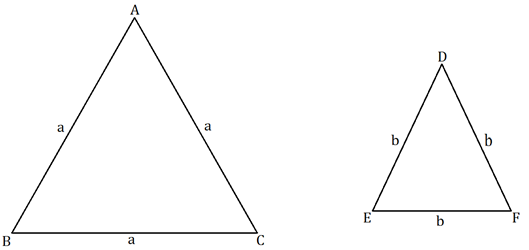ii. If angles in a linear pair are congruent then each of them is a right angle.

Antecedent: Angles in a linear pair are congruent.

Consequence: Each of the angles is a right angle.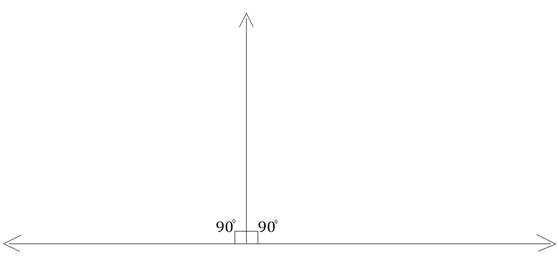iii. If the altitudes drawn on two sides of a triangle are congruent then those two sides are congruent.

Antecedent: The altitudes drawn on two sides of a triangle are congruent.

Consequence: Those two sides are congruent.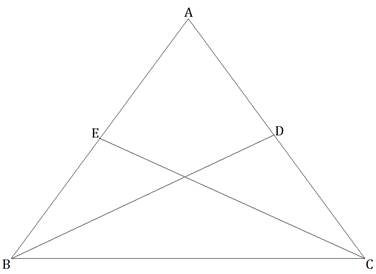## PDF FILE TO YOUR EMAIL IMMEDIATELY PURCHASE NOTES & PAPER SOLUTION. @ Rs. 50/- each (GST extra)

SUBJECTS

HINDI ENTIRE PAPER SOLUTION

MARATHI PAPER SOLUTION
SSC MATHS I PAPER SOLUTION
SSC MATHS II PAPER SOLUTION
SSC SCIENCE I PAPER SOLUTION
SSC SCIENCE II PAPER SOLUTION
SSC ENGLISH PAPER SOLUTION
SSC & HSC ENGLISH WRITING SKILL
HSC ACCOUNTS NOTES
HSC OCM NOTES
HSC ECONOMICS NOTES
HSC SECRETARIAL PRACTICE NOTES

2019 Board Paper Solution

HSC ENGLISH SET A 2019 21st February, 2019

HSC ENGLISH SET B 2019 21st February, 2019

HSC ENGLISH SET C 2019 21st February, 2019

HSC ENGLISH SET D 2019 21st February, 2019

SECRETARIAL PRACTICE (S.P) 2019 25th February, 2019

HSC XII PHYSICS 2019 25th February, 2019

CHEMISTRY XII HSC SOLUTION 27th, February, 2019

OCM PAPER SOLUTION 2019 27th, February, 2019

HSC MATHS PAPER SOLUTION COMMERCE, 2nd March, 2019

HSC MATHS PAPER SOLUTION SCIENCE 2nd, March, 2019

SSC ENGLISH STD 10 5TH MARCH, 2019.

HSC XII ACCOUNTS 2019 6th March, 2019

HSC XII BIOLOGY 2019 6TH March, 2019

HSC XII ECONOMICS 9Th March 2019

SSC Maths I March 2019 Solution 10th Standard11th, March, 2019

SSC MATHS II MARCH 2019 SOLUTION 10TH STD.13th March, 2019

SSC SCIENCE I MARCH 2019 SOLUTION 10TH STD. 15th March, 2019.

SSC SCIENCE II MARCH 2019 SOLUTION 10TH STD. 18th March, 2019.

SSC SOCIAL SCIENCE I MARCH 2019 SOLUTION20th March, 2019

SSC SOCIAL SCIENCE II MARCH 2019 SOLUTION, 22nd March, 2019

XII CBSE - BOARD - MARCH - 2019 ENGLISH - QP + SOLUTIONS, 2nd March, 2019

HSC Maharashtra Board Papers 2020

(Std 12th English Medium)

HSC ECONOMICS MARCH 2020

HSC OCM MARCH 2020

HSC ACCOUNTS MARCH 2020

HSC S.P. MARCH 2020

HSC ENGLISH MARCH 2020

HSC HINDI MARCH 2020

HSC MARATHI MARCH 2020

HSC MATHS MARCH 2020

SSC Maharashtra Board Papers 2020

(Std 10th English Medium)

English MARCH 2020

HindI MARCH 2020

Hindi (Composite) MARCH 2020

Marathi MARCH 2020

Mathematics (Paper 1) MARCH 2020

Mathematics (Paper 2) MARCH 2020

Sanskrit MARCH 2020

Important-formula

THANKS